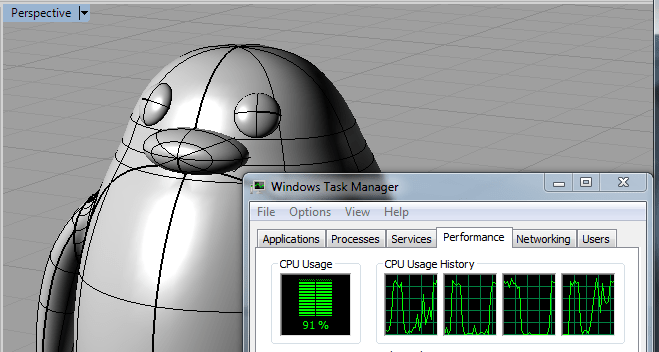Skip to content[
One of the nice bits that we have access to in Rhino python is the Task Parallel Library that is built into .NET 4
http://msdn.microsoft.com/en-us/library/system.threading.tasks.aspx

This set of classes and functions makes it relatively easy to write things like parallel for loops in which every iteration of the loop may be processed on different threads. This nice thing about parallel for loops is that they make coding with multiple threads much simpler since the “multi-threading” only occurs inside the for loop and once the loop is finished you know that the all of the threads have completed and you are back on the main execution thread.

Here’s a sample python script which runs many Plane-Brep intersections either on a single thread or using multiple threads.

 import System.Threading.Tasks as tasks import Rhino import rhinoscriptsyntax as rs import time, math import scriptcontext def radial_contour(brep, parallel, slice_count=360): """Generate series of curve slices through a brep by rotating a plane multiple times and intersecting that plane with the brep. This function demonstrates the use of .NET Parallel.For in order to run the function in parallel Parameters: brep = the Brep to contour parallel = If True, this function will compute intersections in multiple threads using Parallel.For. If False, all intersections will be performed on a single thread slice_count = number of slices to generate. Slices are evenly distributed over a full circle """ if not brep: return results = range(slice_count) rotation_axis = Rhino.Geometry.Vector3d(0,1,0) intersect_tol = scriptcontext.doc.ModelAbsoluteTolerance # local function that does the intersection work. This function is called # once for each angle in "slice_count" and needs to be thread-safe def slice_brep_at_angle(i): try: angle_rad = i/slice_count * 2.0 * math.pi plane = Rhino.Geometry.Plane.WorldXY plane.Rotate(angle_rad, rotation_axis, Rhino.Geometry.Point3d.Origin) rc, crvs, pts = Rhino.Geometry.Intersect.Intersection.BrepPlane(brep, plane, intersect_tol) if rc: results[i] = crvs else: results[i] = None except: pass if parallel: tasks.Parallel.ForEach(xrange(slice_count), slice_brep_at_angle) else: for i in xrange(slice_count): slice_brep_at_angle(i) return results if __name__=="__main__": brep = rs.GetObject("Select Brep", rs.filter.polysurface) brep = rs.coercebrep(brep) if brep: # Make sure the Brep is not under the control of the document. This is # just done so we know we have a quick to access local copy of the brep # and nothing else can interfere while performing calculations brep.EnsurePrivateCopy() #run the function on a sinlge thread start = time.time() slices1 = radial_contour(brep, False) end = time.time() print "serial = ", end–start #run the function on mulitple threads start = time.time() slices2 = radial_contour(brep, True) end = time.time() print "parallel = ", end–start if slices2: for curveset in slices2: if curveset: for curve in curveset: scriptcontext.doc.Objects.AddCurve(curve) scriptcontext.doc.Views.Redraw()

view raw
radial_contour.py
hosted with ❤ by GitHub